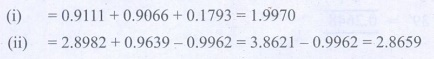Home | | Maths 9th std | Exercise 6.4: Method of using Trigonometric Table

# Exercise 6.4: Method of using Trigonometric Table

Maths : Trigonometry: Book Back, Exercise, Example Numerical Question with Answers, Solution: Exercise 6.4: Method of using Trigonometric Table

Method of using Trigonometric Table

Exercise 6.4

1. Find the value of the following:

(i) sin 49° (ii) cos74°39′ (iii) tan54°26′ (iv) sin21°21′ (v) cos33°53′ (vi) tan70°17′2. Find the value of θ if

(i) sin θ = 0.9975 (ii) cos θ = 0.6763 (iii) tan θ = 0.0720 (iv) cos θ = 0.0410 (v) tan θ = 7.59583. Find the value of the following:

(i) sin 65°39′ + cos 24°57′ + tan10°10′ (ii) tan 70°58′ + cos15°26′ - sin 84°59′4. Find the area of a right triangle whose hypotenuse is 10cm and one of the acute angle is 24° 24′5. Find the angle made by a ladder of length 5m with the ground, if one of its end is 4m away from the wall and the other end is on the wall.6. In the given figure, HT shows the height of a tree standing vertically. From a point P, the angle of elevation of the top of the tree (that is P ) measures 42° and the distance to the tree is 60 metres. Find the height of the tree.Tags : Numerical Problems with Answers, Solution | Trigonometry | Maths , 9th Maths : UNIT 6 : Trigonometry
Study Material, Lecturing Notes, Assignment, Reference, Wiki description explanation, brief detail
9th Maths : UNIT 6 : Trigonometry : Exercise 6.4: Method of using Trigonometric Table | Numerical Problems with Answers, Solution | Trigonometry | Maths